> 文档中心 > Python数学基础二、利用正弦sin求曲边图形的面积

# Python数学基础二、利用正弦sin求曲边图形的面积

## 正弦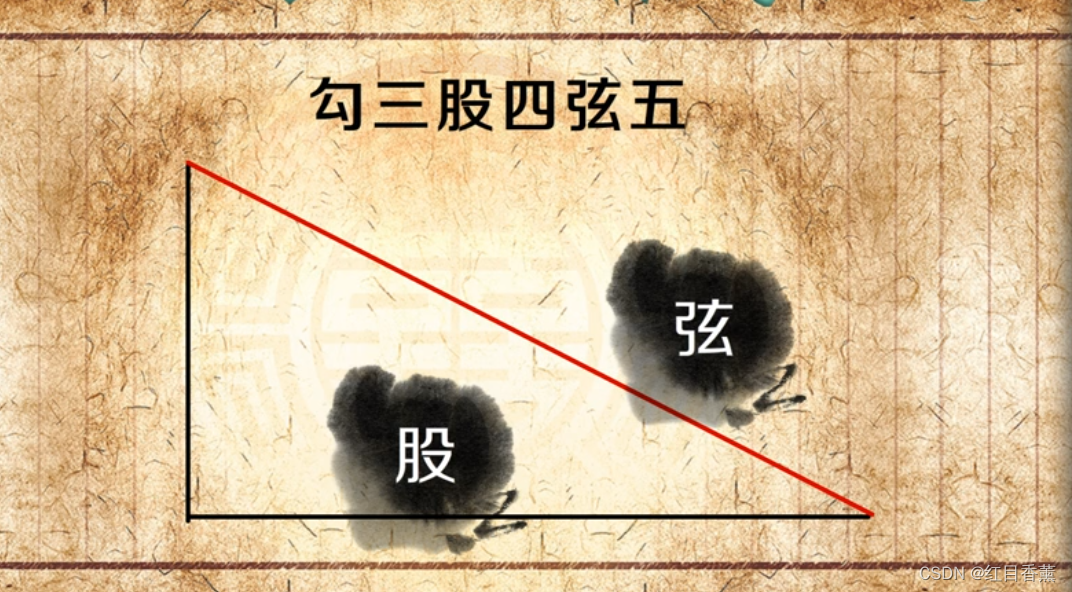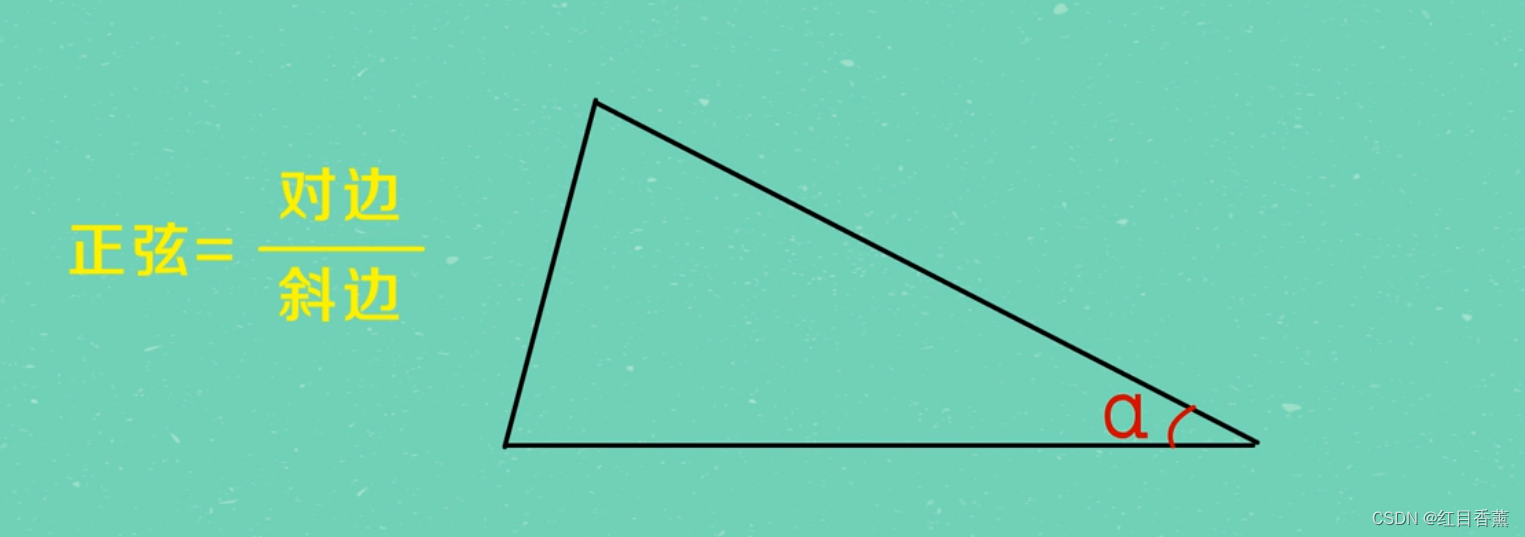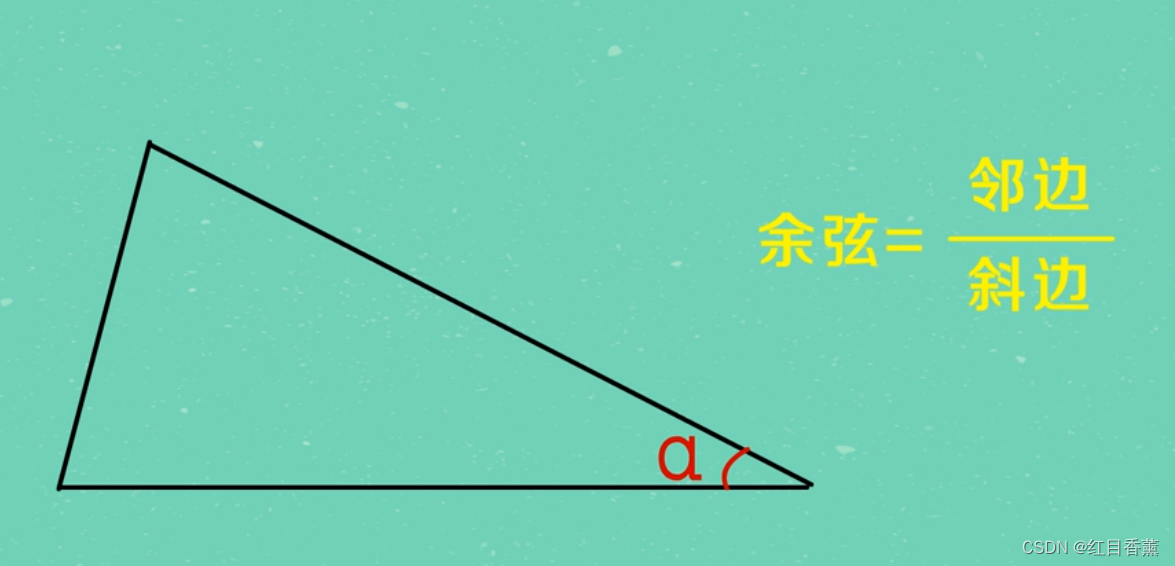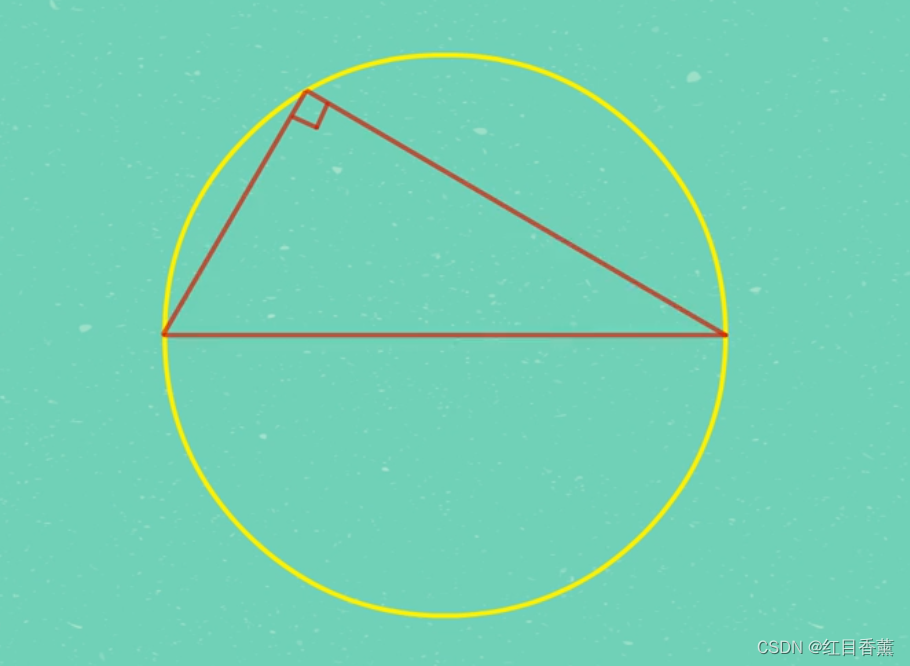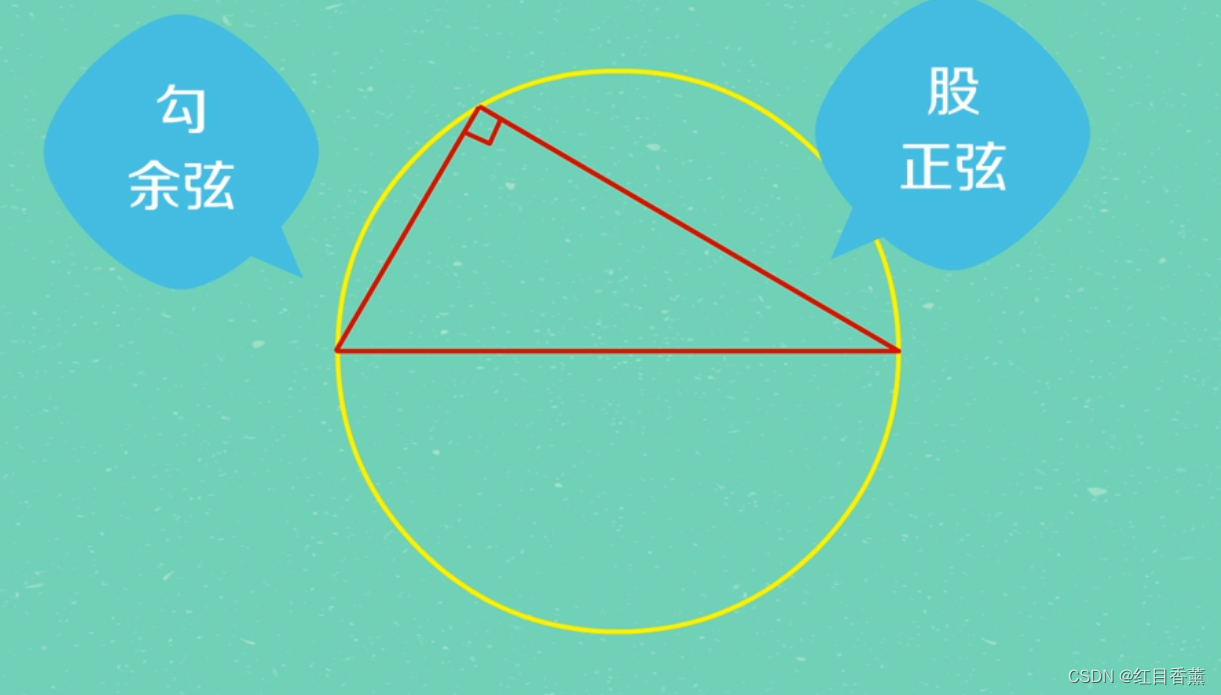∠α的正弦=对边/斜边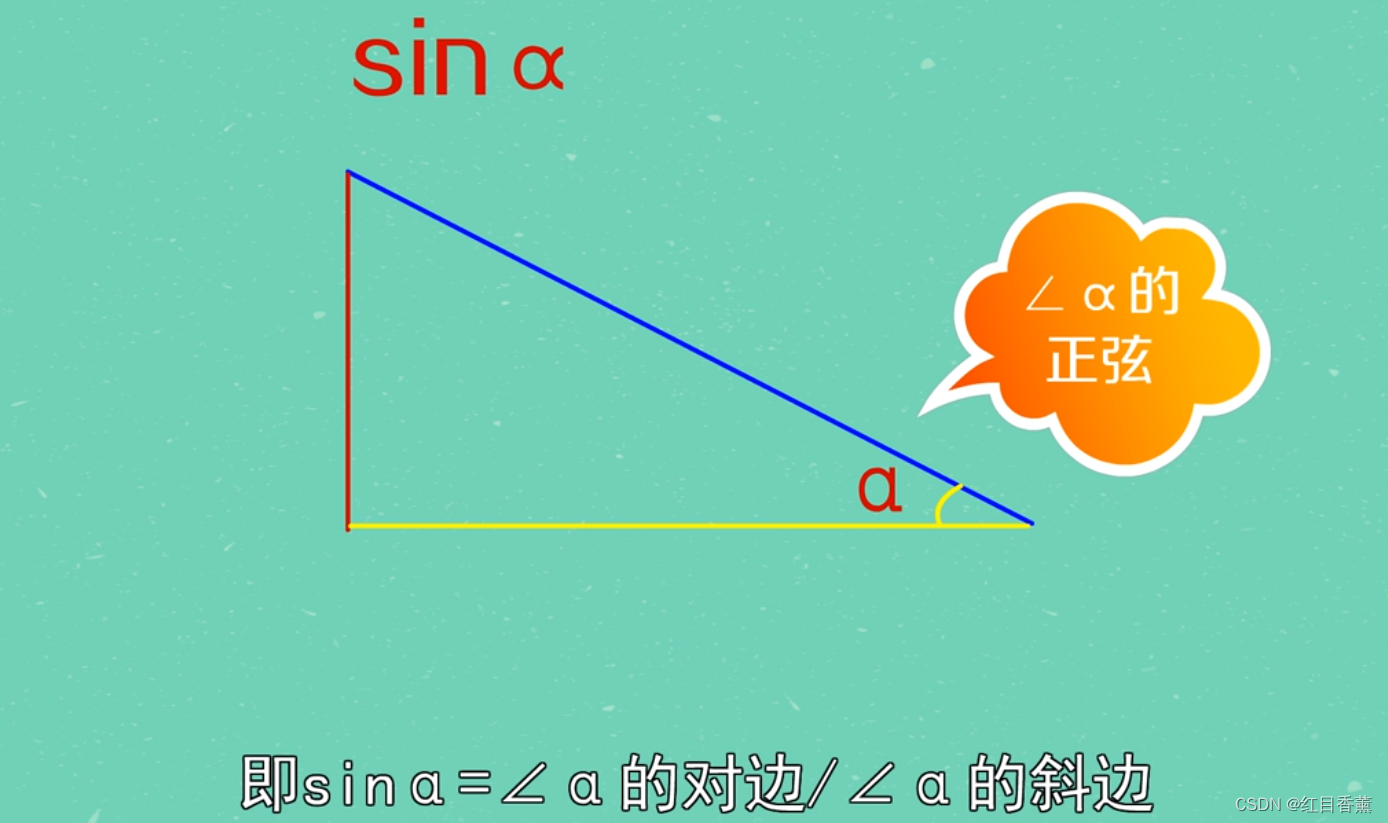## 求曲边图形的面积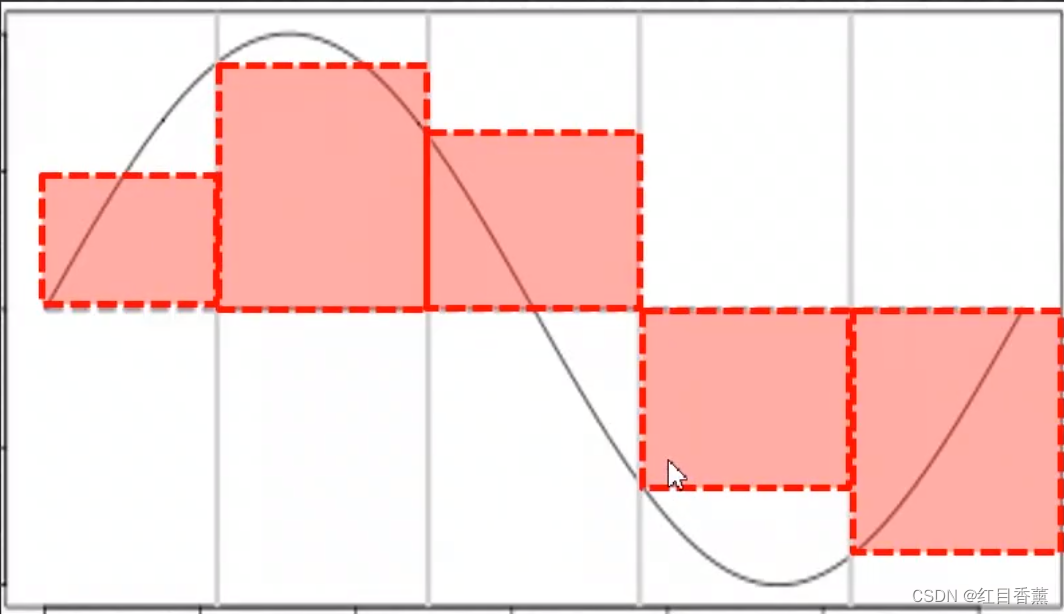1.将各小矩形的高度存放至一列表中。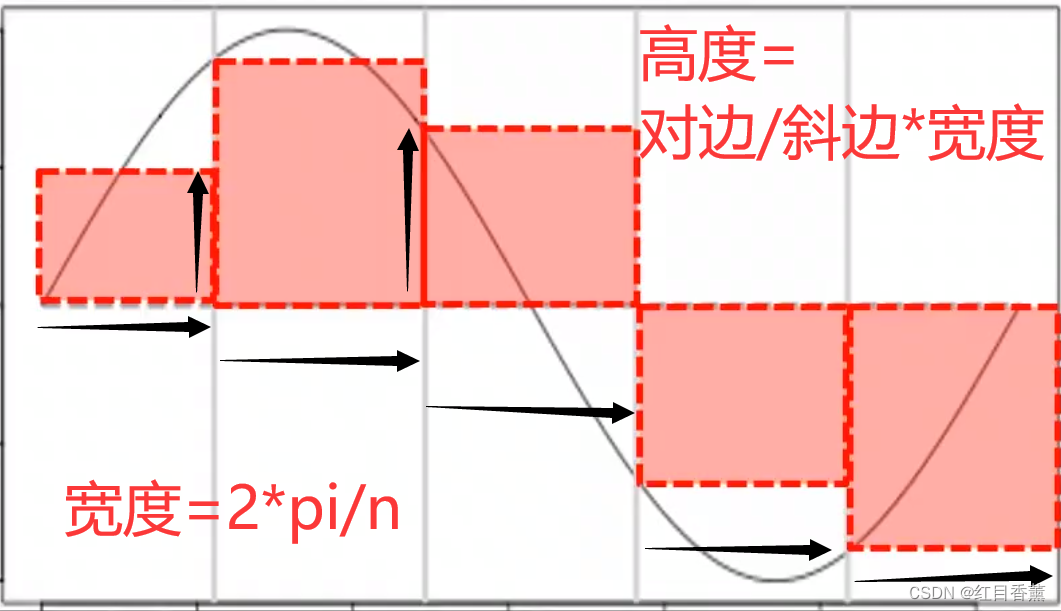2.将各高度乘以宽度，得各矩形面积。这里可以分为无数的小矩形。

3.求和。

sum(叫矩形面积数组)

## 推导方式解法：

``# 求曲边图形的面积import math# 先拆分10个简单算一下。不精确n = 10# 每个宽度=2*pi/nwidth = 2 * math.pi / n# 宽度数组x = []# 把x轴每次延伸的值逐一放进去for i in range(n):    x.append((i * width))# 高度数组y = []# 遍历宽度，根据高度=正弦的绝对值，由于是正弦肯定有正负，那么我们就计算一下绝对值。for i in x:    y.append(abs(math.sin(i)))# 求和S = sum(y) * widthprint(S)``

## 推导式解法：

``# 求曲边图形的面积import math# 先拆分10个简单算一下。不精确n = 10# 每个宽度=2*pi/nwidth = 2 * math.pi / n# 推导式s = [abs(math.sin(i * width)) * width for i in range(n)]print(sum(s))``

KTV音响网